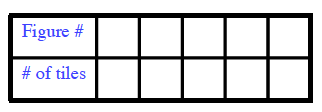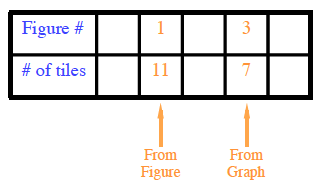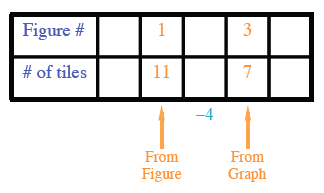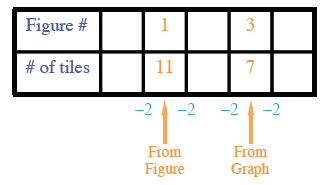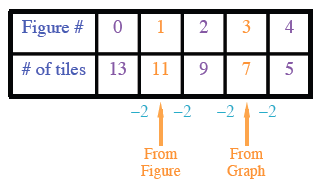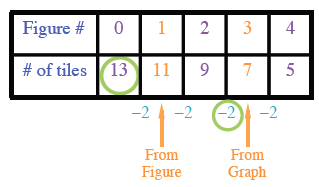Home > CC4 > Chapter 3 > Lesson 3.1.3 > Problem3-34

3-34.

Write an equation for the tile pattern represented at right. Homework Help ✎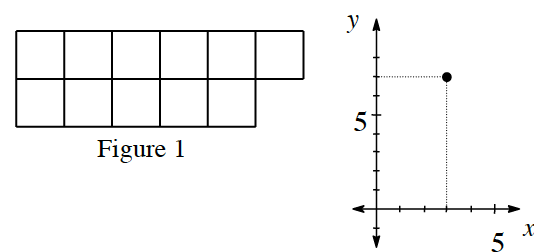Create a table to help find a pattern.

Fill in the information that you are given.

What is the difference between the number of tiles in the two figures?

See Table. Fill in figure numbers.

Use this information to find the difference between the number of tiles in consecutive figures.

See Table

Now fill in the rest of the table.

Using the starting point and the growth rate,write an equation to represent the pattern.